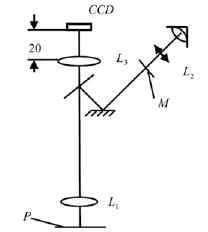# Geometric optics, microscope

• Grufey

## Homework Statement

Let us consider a microscope where the objective L1 has f1=20mm and magnification 10x. In the image plane is located a diafragm M with diameter 19mm (see fig). The size of the CCD is 4,8mm (vertical) x 5,6mm (horizontal). 20mm before of the CCD camera a len L3 is introduced in order to obtain the best compatibility of the field in the both paths of the microscope. Assumins that the focal length of the eyepiece L2 is 25 mm, determine:

a) Distance Object-Objective
b) Diameter of the observation field
c) Overal magnification in the direct observation (eye) path## Homework Equations

General geometric optics:
Magnification: m=-y'/y where y and y' are the height of the object and image respectively
Lens formula: 1/s+1/s'=1/f where s and s' are object and image distance respectively and f the focal length

It should not need more formula, I guess...

## The Attempt at a Solution

My attempt of solution begins with the lens formula applied to the Len L1

$\frac{1}{s}+\frac{1}{s'}=\frac{1}{f}$ then, multiplying by s', we get, $m=\frac{s'}{s}=\frac{s'-f}{f}$

Thus substituting m=10,f=20, we get s'=220 mm, now applying again, the lens formula, we get s=-22mm. The minus sign denotes, that the object is in the object space. Ok until here everything is undertood, isn't it?

My problen is the b) and c) part. I think I need more information about L2. How can I get the magnification and the diameter in the observation field?

On the magnification, I think it means size magnification, or it is angular magnification?

Thanks in adavance, any help will be useful

(b)
My understanding is that they want to know the diameter of the field on the object table that can be observed through the diaphragm. Not so hard if you did the calculation for the magnification factor object plane - image plane.

(c)
L2 has a focal length of 25 mm. Optical power 40 diopter. If I can normally focus to 25 cm, its magnification is ... (however, one less if you want to look with an unaccommodated eye, i.e. with the ocular focused on the image plane)
For a microscope the total magnification is ...

Caveat: I'm no expert, but I wear glasses and do possesses a microscope. Gave it my best shot, but it's your exercise, so if you don't understand or don't agree then don't take my word for it :)

Re your last question: I would stick to linear magnification. But Wiki (on the same page here ) leans the other way:
By convention, for https://www.physicsforums.com/wiki/Magnifying_glass [Broken] and optical https://www.physicsforums.com/wiki/Microscope [Broken], where the size of the object is a linear dimension and the apparent size is an angle, the magnification is the ratio between the apparent (angular) size as seen in the eyepiece and the angular size of the object when placed at the conventional closest distance of distinct vision: 25 cm from the eye.

Last edited by a moderator: